# How to write a vector in LaTeX?

When you work online and when you create a science-related document, you need to know about latex if you want to use vector equations in that document.

There can be different types of documents. Examples are science books, research papers, online science content, and science notes, etc.

In this tutorial, we will discuss in-depth how to use latex to represent different types of vectors.

## How to identify vectors with the help of latex?

The vector is always denoted by an arrow on the specified character. Here the character can be one or more.

For example, you take a vector whose initial points and final points are a and b. And you named the vector p. In this case, you can call the vector both p and ab.

You see, here ab is made up of more than one character.

So, you have to use the \vec and \overrightarrow commands to vector arrow on a character in latex. And you have to pass that character as an argument in this command. Suppose I write p as a vector here.

\documentclass{article}
\begin{document}
$$\vec{p}$$
\end{document}

Output :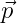You all know about momentum which is a vector quantity. And this is the product of mass and velocity.

\documentclass{article}
\begin{document}
$$\vec{p}=m\vec{v}$$
\end{document}

Output :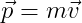Suppose a particle travels from point A to point B, then the displacement of a particle will be

\documentclass{article}
\begin{document}
$$\vec{AB}$$
\end{document}

Output :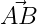You notice the output above, the vector arrow does not cover the head of the whole AB. In this case, you need to use the \overrightarrow command instead of the \vec command.

\documentclass{article}
\begin{document}
$$\overrightarrow{AB}$$
\end{document}

Output :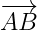Therefore, it is best to use the \overrightarrow command when denoting a vector by more than one character.

Also, you will notice that in many books or research papers vectors are marked in bold instead of an arrow.

\documentclass{article}
\begin{document}
$$\textbf{AB}$$
\end{document}

Output :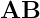That is, you need to use the \textbf  command whenever you want to make a text bold.

## Magnitude of a vector in latex

When the magnitude of the vector is determined. Then the vector is denoted in mod. So, in this case, vectors have to pass between \left | and \right | as arguments.

\documentclass{article}
\begin{document}
$$\left| \vec{a} \right|$$
\end{document}

Output :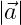Latex has many packages that you can install and use the mod easily. We have discussed here the simple method without installing any external package.

## Special type of vector in latex

There are different types of vectors. Such as unit vectors, zero vectors which are denoted separately.

### 1. Unit Vector

The unit vector is denoted by placing a cap on a single character. And dividing a vector by the absolute value of that vector defines the unit vector. For example

\documentclass{article}
\begin{document}
$$\hat{a}=\frac{\vec{a}}{\left | \vec{a} \right |}$$
\end{document}

Output :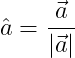Notice the program above. To put a cap on a character, you need to pass that character in the \hat command. And for fraction, you have to use \frac command in which you have to pass two arguments as numerator and denominator.

### 2. Zero Vector

And for the absolute value of the vector, you know what to use as discussed in the previous point.

If the absolute value of the vector is zero then it is called a null vector or zero vector. Which is denoted with an arrow sign above zero.

Suppose the total applied force on a system is zero. In that case, the force has to be expressed in the form of a zero vector.

\documentclass{article}
\begin{document}
$$\vec{F}_{net}=\vec{0}$$
\end{document}

Output :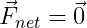## Position Vector

A position vector is represented by three rectangular unit vectors.

\documentclass{article}
\begin{document}
$$\vec{r}=x\hat{i}+y\hat{i}+z\hat{i}$$
\end{document}


Output :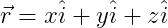And the \hat command is called to cap the head of each unit vector.

## Vector Multiplication

Two types of products are usually seen between two vectors.

### 1. Cross Product in LaTeX

The cross product is denoted by a cross mark between two vectors. And its result is also a vector that is written in the form of a unit vector or matrix. And you need to use the \times command to use the cross mark.

\documentclass{article}
\begin{document}
$$\vec{p}\times \vec{q}=|\vec{p}|\vec{q}|sin\theta \hat{n}$$
\end{document}

Output :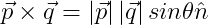For theta, you need to use the \theta command. In which no argument has to be passed.

### 2. Dot Product in LaTeX

You can use dot symbols for dot products in different ways. We will use the \cdot command for vector dot product.

\documentclass{article}
\begin{document}
$$\vec{p}\cdot\vec{q}=|\vec{p}|\vec{q}|cos\theta$$
\end{document}

Output :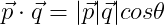## Use physics package for vector in LaTeX

In latex, the physics package is defined for each special symbol or operation of the physics. For example, vector arrows and vector bold are denoted by va and vb commands.

\documentclass{article}
\usepackage{physics}
\begin{document}
$$\va{a}$$
$$\va*{a}$$
$$\vb{a}$$
$$\vb*{a}$$
\end{document}

Output :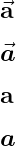And you can easily represent all kinds of vector operations with the help of physics package. Because the physics package contains pre-defined commands or functions to denote these operations. For example

\documentclass{article}
\usepackage{physics}
\begin{document}
$$\vu*{a}$$
$$\va{p}\vdot\va{q}$$
$$\va{p}\cp\va{q}$$
$$\abs{\va*{a}}$$
\end{document}

Output :#### Md Jidan Mondal

LaTeX expert with over 10 years of experience in document preparation and typesetting. Specializes in creating professional documents, reports, and presentations using LaTeX.